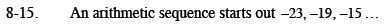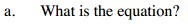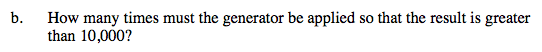Home > CCA2 > Chapter 8 > Lesson 8.1.1 > Problem8-15

8-15.
1. An arithmetic sequence starts out −23,−19,−15… Homework Help ✎

1. What is the equation?

2. How many times must the generator be applied so that the result is greater than 10,000?What is the common difference between the terms?

Remember that the first term is -23. What is the 0th term?Use the rule you found in part (a).